### Dependent Types and Multi-monadic Effects in F*

N. Swamy, C. Hritcu, C. Keller, A. Rastogi, S. Forest,
A. Delignat-Lavaud, K. Bhargavan, C. Fournet, P. Strub,
M. Kohlweiss, J. Zinzindohoue, S. Zanella-Beguelin

Microsoft Research, MSR-INRIA, INRIA, UMD, IMDEA

### Shall the twain ever meet?

The two, mostly disjoint sides of program verification research

Interactive proof assistants Semi-automated verifiers of imperative programs
Coq, CompCert,   air Dafny, Verve,
Agda, Bedrock, Vcc, miTLS
Lean, Feit-Thompson   gap Verifast,
• In the left corner: Arbitrarily expressive logics, but only purely functional programming

• In the right: Only first-order logic, but rich, effectful programming

### Bridging the gap: F*

• A general purpose, strict, higher-order effectful language

• like ML, with a predictable CBV cost model
• An SMT-based, semi-automated program verification tool,

• like Dafny, Why3 etc.
• On a foundation of dependent and inductive types

• like Coq, Agda etc.
• Also in the gap: ATS, HTT, Idris, Trellys,

• But none give you all three

### Illustrating the threefold nature of F*

• F* is programmed in F* and bootstraps in OCaml or F#

• We use it daily for general-purpose programming
• Verified implementations of (parts of) the TLS protocol

• Relying on SMT for verifying effectful programs
•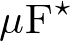is (partially) formalized in F*

• We use it as a proof assistant (at least for PL metatheory)

### A first example: Quicksort

let rec quicksort f = function
| [] -> []
| pivot::tl -> let hi, lo = partition (f pivot) tl in
quicksort f lo @ pivot::quicksort f hi

• Total correctness of quicksort, proven via a combination of F* type-checking and SMT solving

  val quicksort: f:total_order a
-> l:list a
-> Tot
(m:list a{sorted f m /\ forall i. count i l = count i m})
(decreases (length l)) 
• Plus some quicksort-specific lemmas about partition and @

val quicksort: f:total_order a
-> l:list a
-> Tot
(m:list a{sorted f m /\ forall i. count i l = count i m})
(decreases (length l)) 

### Semantic termination checking

val quicksort: f:total_order a
-> l:list a
-> Tot //Total function: semantic termination checking
(m:list a{sorted f m /\ forall i. count i l = count i m})
(decreases (length l)) //termination metric
• From library:

let rec length = function [] -> 0 | _::tl -> 1 + length l

### Full dependency and refinement types

val quicksort: f:total_order a
-> l:list a
-> Tot (m:list a{sorted f m /\ forall i. count i l = count i m})
(* ^^^^^^^^^^^refinement types^^^^^^^^^^^^^^^ *)
• From library:

let rec sorted f = function
| [] | [_] -> true
| x::y::tl -> f x y && sorted f (y::tl)

let rec count i = function
| [] -> 0
| hd::tl when i=hd -> 1 + count tl
| _::tl -> count tl

### Refinements at higher order

val quicksort: f:total_order a //refinements at higher order
-> l:list a
-> Tot (m:list a{sorted f m /\ forall i. count i l = count i m})
• From library:

type total_order a = f:(a -> a -> Tot bool){
(forall a. f a a)                                           (* reflexivity   *)
/\ (forall a1 a2. f a1 a2 /\ f a2 a1  ==> a1 = a2)          (* anti-symmetry *)
/\ (forall a1 a2 a3. f a1 a2 /\ f a2 a3 ==> f a1 a3)        (* transitivity  *)
/\ (forall a1 a2. f a1 a2 \/ f a2 a1)                       (* totality  *)
}

### Doesn't sound like the F* you've heard about before?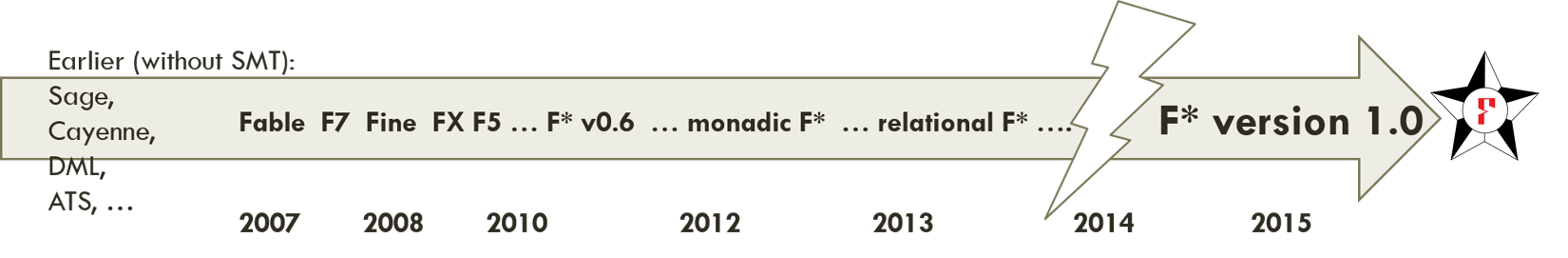• Open source, binaries for all platforms

• Full dependent types with inductive families and refinements

• Primitive support for effects, specified using a lattice of predicate-transformer monads

• Type and effect inference

• SMT for large boring proofs

• Dependently typed programs as proofs, when SMT fails

• Semantic termination checking

### Rest of the talk

• Primitive effects specified using a lattice of Dijkstra monads

• Fragments of the formalization and main theorems

### Programming with primitive effects, like in ML

• Divergence:

let server () = let req = get () in ...; server ()
• Exceptions:

let rec find f = function
| [] -> raise Not_found
| hd::tl -> if f hd then hd else find f tl
• State:

let ctr =
let i = ref 0 in
fun () -> incr i; !i

### Indexed computation types track effects

• Unconditionally pure: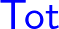1 + 1       : Tot int  
• Conditionally pure: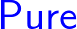factorial x : Pure int (requires (x >= 0)) (ensures (fun y -> y >= 0)) 
• By subtyping:

type nat = x:int{x >= 0}
factorial 1 : Tot nat
• Stateful: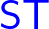x := 17     : ST unit (requires (fun h -> h contains x))
(ensures (fun h0 _ h1 -> h1 = upd h0 x 17))

### Effect inclusion via a user-defined lattice

• Effect inclusion:is lifted to1 + 1       : ST int (requires (fun h -> True))
(ensures (fun h0 x h1 -> x=1+1 /\ h0=h1))
• A lattice of effect orderings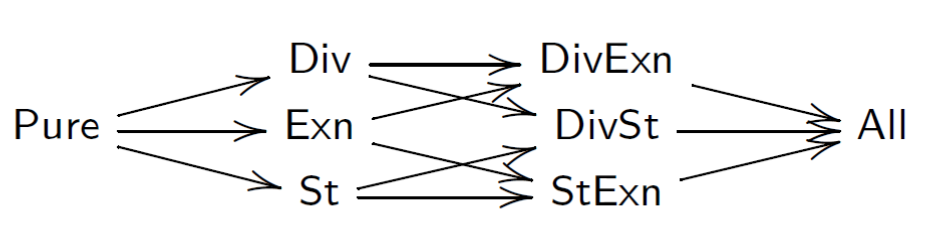• Can also pick other effects (e.g., Ghost, IO, NonDet, )

### Structuring the effect lattice

• Each point in the lattice is a monad of predicate transformers (aka a Dijkstra monad)

• Key idea, in three steps:

1. Fix the bottom and top of the lattice:.wp and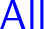.wp

2. In between, partition the effects as needed, defining a WP calculus for each sub-effect and monad morphisms to partially order effects

• E.g.,.wp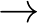.wp.wp
3. The monad morphism laws ensure that any user-defined effect is compatible with the primitive semantics provided by F*'s.wp monad

### Structuring the monad lattice, by example

•.wp: a monad for pure computations at the bottom

Pure.post a = a -> Type
Pure.pre    = Type
Pure.wp a   = Pure.post a -> Pure.pre
Pure.return (x:a) : Pure.wp a  = fun post -> post x
Pure.bind (wp1:Pure.wp a) (wp2: a -> Pure.wp b) : Pure.wp b =
fun post -> wp1 (fun x -> wp2 x post)
•.wp: a monad for all computations at the top

All.post a = mem -> (a + exn) -> Type
All.pre    = mem -> Type
All.wp a   = All.post a -> All.pre
All.return (x:a) : All.wp a  = fun post mem -> post mem (Inl x)
All.bind (wp1:All.wp a) (wp2:a -> All.wp b) : All.wp b =
fun post m0 -> wp1 (fun m1 -> function
| Inl x  -> wp2 x post m1
| Inr ex -> post m1 (Inr ex)) m0

###.wp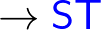.wp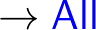.wp

ST.post a = mem -> a -> Type
ST.pre    = mem -> Type
ST.wp   a = ST.post a -> ST.pre
ST.return x = fun post m0 -> post m0 x.
ST.bind wp1 wp2 = fun post m0 -> wp1 (fun m1 x -> wp2 x m1 post) m0
• Monad morphisms witnessing effect inclusion

lift_pure_st : Pure.wp a -> ST.wp a = fun wp post m0 -> wp (post m0)
lift_st_all  : ST.wp a -> All.wp a = fun wp post -> wp (fun m1 x -> post m1 (Inl x))
• Lifts must commute (per the morphism laws), to ensure the user-provided semantics ofcoincides with F*'s built-in semantics of state within. For example:

    All.bind (lift_st_all wpa) (lift_st_all . wp') =ext= lift_st_all (ST.bind wp wp')

### User-defined abstract memory layout: E.g., regions

• Beyond simply refining the effect lattice, F* also allows you to choose alternative memory representations

• Paper gives a region-inspired memory model for expressing heap separation invariants while remaining within SMT
• Need to prove that your custom memory layout can be realized on the flat primitive heap

### Benefits of our multi-monadic approach

• Flexibility in structuring the effects

• Type inference is built-in via the WP calculus, with automatic lifting of effects as needed

• Modular specifications and complexity of VCs reflecting only the effects that are actually used

• e.g., don't have to reason needlessly about exponentially many paths due to exceptions that we know will never be thrown in code that is syntactically exception-free

### Formalizing all of F*: A work in progress

Two subsets formalized so far:

•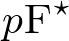: A pure core of F*, with a built-in WP calculus, fixpoints and semantic termination checking

• Weak-normalization (CBV), via logical relations
• Logical consistency: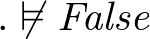•: CBV calculus with dependent types, non-termination and state, user-defined Dijkstra monad lattice and memory layout, proven partially correct

• For a well-typed configuration: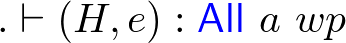such that its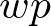is valid for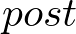: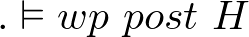• Then, either,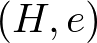diverges
• Or,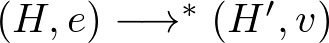and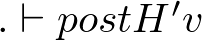### Currently underway

• A verified implementation of TLS-1.2 and TLS-1.3 with a joint security theorem

• Beyond the primitive-effect: Extending F* with user-defined non-primitive effects

• Developing F* further as a proof assistant: An Mtac-inspired tactic language

### Conclusion

• Many semi-automated verifiers for first-order languages

• Relatively simple logics, effectful, good SMT automation
• Many interactive proof assistants

• Good control, but automation lacking; effects via encoding
• F* tries to span the divide

• Higher order with customizable, primitive effects
• SMT for large boring proofs; dependently typed programs as proofs, when SMT fails
• We're hiring! PhD students, interns, post-docs, researchers
• Find Catalin Hritcu or me